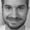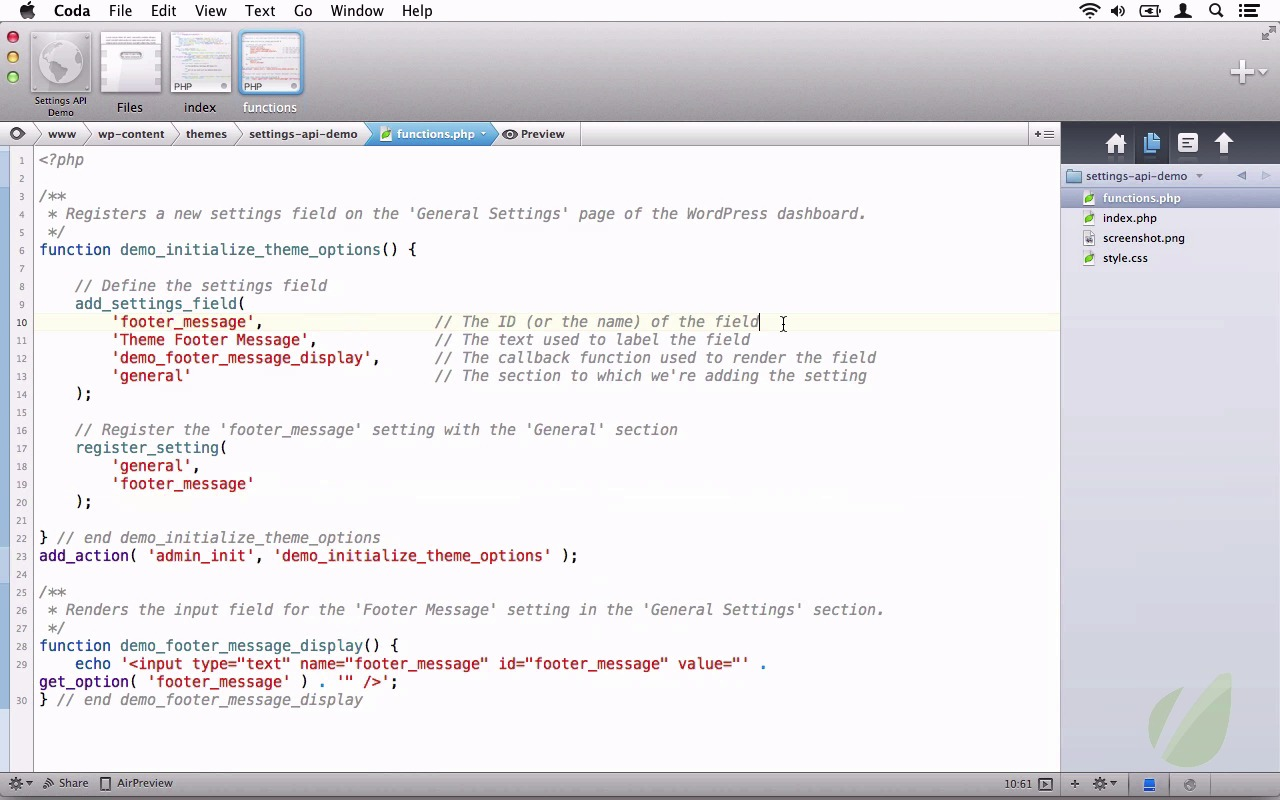Lessons: 15Length: 2.2 hours• Overview
• Transcript

# 2.4 Section, Fields and Settings

The point of this lesson is to develop a deep understanding the relationship between the three entities in the context of the Settings API. This is a critical component in order to move forward with the series, so there will be a discussion on each and how they relate to one another.

In the lesson, we’ll do the following:

• Take a look at each entitiy and how they relate to each other
• Take a survey of the entities
• Then we’ll look at each function
Resources